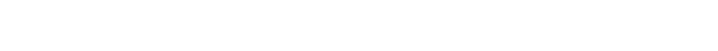Published on

# Concise Computation of Subsets in Haskell

I recently discovered one of the most elegant Haskell functions I’ve ever encountered on StackOverflow. So, I decided to write about it – just for fun. Also, I’m trying to convince my boss, who is a Ruby guy, to give Haskell a shot :)

The function’s original name is `choose` and its purpose is the computation of all subsets with size `k` from a given superset.

First off, here’s the code from StackOverflow:

``````choose :: [b] -> Int -> [[b]]
_      `choose` 0 = [[]]
[]     `choose` _ =  []
(x:xs) `choose` k =  (x:) `fmap` (xs `choose` (k-1)) ++ xs `choose` k``````

``````module Combinations (kCombinations) where

kCombinations :: [a] -> Integer -> [[a]]
kCombinations _      0 = [[]]
kCombinations []     _ =  []
where withHead    = fmap (x:) (kCombinations xs (k-1))

## How does it work?

The first argument specifies the superset (i.e. `(x:xs)`) and the second argument (i.e. `k`) the size of the subsets we are looking for.

Now, as you can see, the function calls itself twice:

1. The first call, picks `k-1` elements from the tail of the superset (i.e. `xs`), resulting in a list of subsets with size `k-1`. To each of these, the head of the superset (i.e. `x`) is prepended using the cons operator.
2. The second call, picks all `k` elements from the tail. Therefore, the head of the superset (i.e. `x`) can never be included in one of the subsets resulting from this call.

Finally, we have two edge conditions for the recursion: If …

1. `k == 0`, the result is always `[[]]` – the only subset with size `0` is `[]`, which is obviously true for all conceivable supersets.
2. … the superset is empty and we are looking for subsets with `k > 0` elements, the result is always `[]`. This is true by definition, because subsets can never contain more elements than the superset.

Just in case my explanation isn’t clear enough, here’s the same function, implemented in vanilla JavaScript:

``````function kCombinations(superset, k) {
if (0 === k)               { return [[]]; }
if (0 === superset.length) { return  [];  }

var x  = superset;       // head of superset
var xs = superset.slice(1); // tail of superset

// pick k-1 elements from tail & prepend head to each:

// pick all k elements from tail:

}``````

## Automated Testing with QuickCheck

As I’ve explained last month, the first thing we need to implement a test with QuickCheck is some kind of universal property the function satisfies. The first one I can think of is the number of subsets `kCombinations` generates. So, let’s go with that.

If we would compute the entire powerset, there would be exactlydistinct subsets. However, since we are only interested in subsets with given size `k`, there must be exactlydistinct subsets. This formula is well known in combinatorics. And, expressing it in Haskell is straightforward:

``````import Data.List (genericLength)

kCombinationsCardinality :: [a] -> Integer -> Integer
kCombinationsCardinality xs k =
let n = genericLength xs in
let fact m = product [1..m] in
(fact n) `div` ( (fact k) * (fact \$ n-k) )``````

With `kCombinations` and `kCombinationsCardinality` we have everything we need to compose our test:

``````prop_kCombinations :: Positive Integer -> Positive Integer -> Bool
prop_kCombinations (Positive n) (Positive k) = actual == expected
where actual   = genericLength \$ kCombinations [1..n] k
expected = kCombinationsCardinality [1..n] k``````

QuickCheck is repeatedly applying `prop_kCombinations` to randomly generated arguments. Each of these applications, constitutes a separate test-case. `prop_kCombinations` is computing the number of subsets `kCombinations` actually generates and the number of subsets we expect to see. The test-case passes if these two numbers are identical. Otherwise it fails.

Our test is in principal correct, but we are not done yet. The test takes very long to execute, if QuickCheck picks large numbers as arguments. We should probably fix that, unless you enjoy waiting for your test suite to finish.

The solution is to enforce an upper bound for the arguments. To keep things simple, we can use Haskell’s `mod` function. With that being said, the final test looks as follows:

``````{-# LANGUAGE ScopedTypeVariables, TemplateHaskell #-}
module Main where

import Combinations
import Test.QuickCheck
import Test.QuickCheck.All
import Data.List (genericLength)

kCombinationsCardinality :: [a] -> Integer -> Integer
kCombinationsCardinality xs k =
let n = genericLength xs in
let fact m = product [1..m] in
(fact n) `div` ( (fact k) * (fact \$ n-k) )

prop_kCombinations :: Positive Integer -> Positive Integer -> Bool
prop_kCombinations (Positive n) (Positive k) = actual == expected
where n' = n `mod` 20
k' = k `mod` 20
actual   = genericLength \$ kCombinations [1..n'] k'
expected = kCombinationsCardinality [1..n'] k'

return []
main = \$(verboseCheckAll)``````

Notes